Worksheets and No Prep Teaching Resources
Math Worksheets

# Transformations Worksheets

Being able to visualize is a critical skill that gets better with practice, and these exciting worksheets on transformations will ensure that students develop their visual-spatial intelligence. Students will enjoy deciphering how images look with horizontal flips, vertical flips, as well as clockwise and counterclockwise turns. They will have a blast picking the correct images as well as drawing them! Add these worksheets to math curriculums for a refreshing change of pace in the classroom.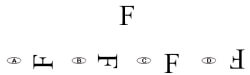Introduction to Horizontal Flips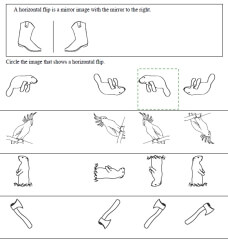Horizontal Flips: Circle the image - first answer given - pictures Horizontal Flips: Circle the image - first answer given - letters and pictures Horizontal Flips: Circle the image - letters and pictures Horizontal Flips: Circle the image - letters and pictures - no instructions with sample at top Horizontal Flips: Circle the image - first answer given - letters

Introduction to Vertical Flips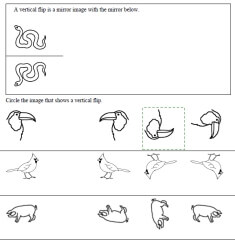Vertical Flips: Circle the image - first answer given - pictures Vertical Flips: Circle the image - first answer given - letters and pictures Vertical Flips: Circle the image - letters and pictures Vertical Flips: Circle the image - letters and pictures - no instructions with sample at top Vertical Flips: Circle the image - first answer given - letters

Introduction to Clockwise Turns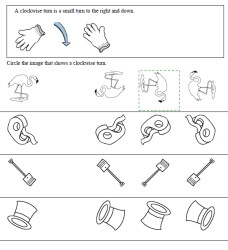Clockwise Turns: Circle the image - first answer given - pictures Clockwise Turns: Circle the image - first answer given - letters and pictures Clockwise Turns: Circle the image - letters and pictures Clockwise Turns: Circle the image - letters and pictures - no instructions with sample at top Clockwise Turns: Circle the image - first answer given - letters

Introduction to Counterclockwise Turns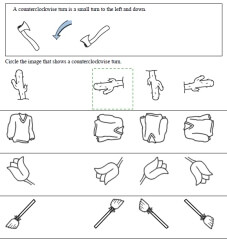Counterclockwise Turns: Circle the image - first answer given - pictures Counterclockwise Turns: Circle the image - first answer given - letters and pictures Counterclockwise Turns: Circle the image - letters and pictures Counterclockwise Turns: Circle the image - letters and pictures - no instructions with sample at top Counterclockwise Turns: Circle the image - first answer given - letters

Drawing Slides, Flips, and Turns
Drawing Slides - Easier (just says to draw a slide, no specific directions)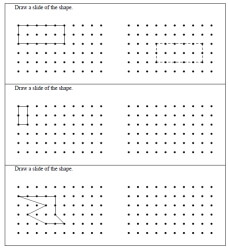Drawing Slides - trace answers given to each Drawing Slides - trace answers given to first problem only Drawing Slides

Drawing Slides - Exact Directions Given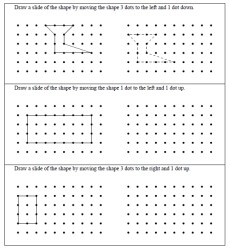Drawing Slides - trace answers given to each Drawing Slides - trace answers given to first problem only Drawing Slides

Drawing Horizontal Flips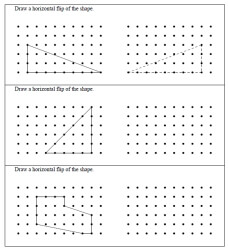Drawing Horizontal Flips - trace answers given to each Drawing Horizontal Flips - trace answers given to first problem only Drawing Horizontal Flips

Drawing Vertical Flips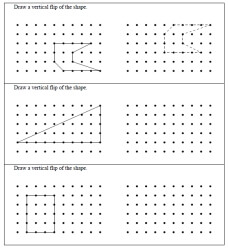Drawing Vertical Flips - trace answers given to each Drawing Vertical Flips - trace answers given to first problem only Drawing Vertical Flips

Drawing Flips - Horizontal and Vertical Flips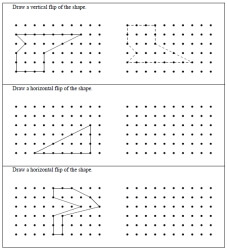Drawing Flips - trace answers given to each Drawing Flips - trace answers given to first problem only Drawing Flips

Drawing Clockwise Turns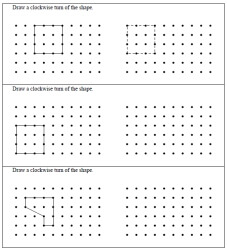Drawing Clockwise Turns - trace answers given to each Drawing Clockwise Turns - trace answers given to first problem only Drawing Clockwise Turns

Drawing Counterclockwise Turns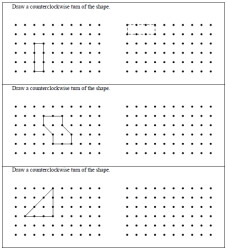Drawing Counterclockwise Turns - trace answers given to each Drawing Counterclockwise Turns - trace answers given to first problem only Drawing Counterclockwise Turns

Drawing Turns - Clockwise and Counterclockwise Turns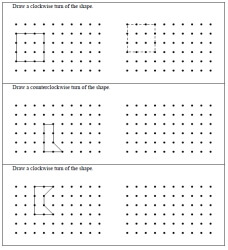Drawing Turns - trace answers given to each Drawing Turns - trace answers given to first problem only Drawing Turns

Drawing Slides, Turns, and Flips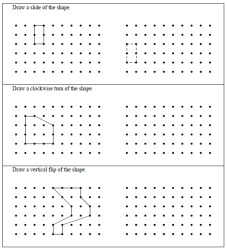Drawing Slides, Turns, and Flips - trace answers given to each Drawing Slides, Turns, and Flips - trace answers given to first problem only Drawing Slides, Turns, and Flips

Flips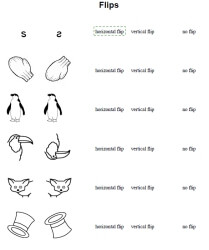Flips - pictures - multiple choice: circle horizontal flip, vertical flip, or no flip Flips - letters or pictures - multiple choice: circle horizontal flip, vertical flip, or no flip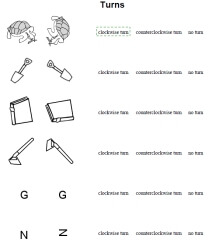Flips - smaller pictures (more problems on a page) - multiple choice: circle horizontal flip, vertical flip, or no flip Flips - smaller letters or smaller pictures (more problems on a page) - multiple choice: circle horizontal flip, vertical flip, or no flip

Turns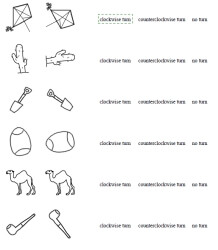Turns - pictures - multiple choice: circle clockwise turn, counterclockwise turn, or no turn Turns - letters or pictures - multiple choice: circle clockwise turn, counterclockwise turn, or no turn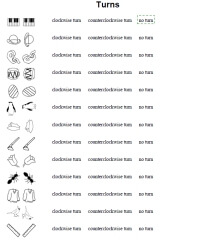Turns - smaller pictures (more problems on a page) - multiple choice: circle clockwise turn, counterclockwise turn, or no turn Turns - smaller letters or smaller pictures (more problems on a page) - multiple choice: circle clockwise turn, counterclockwise turn, or no turn

Flips and Turns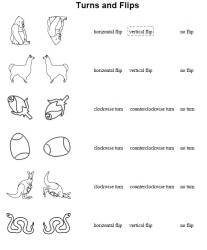Flips and Turns - pictures Flips and Turns - letters or pictures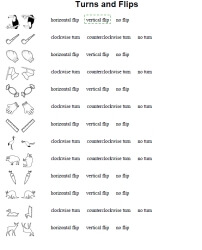Flips and Turns - smaller pictures (more problems on a page) Flips and Turns - smaller letters or smaller pictures (more problems on a page)

Redraw, Enlarge, and Reduce Shapes

Coordinate Graphing
Coordinate Plane and Functions
Write the coordinates for each point
Finding ordered pairs
Drawing ordered pairs
Complete the function table
Complete the function table and then graph the function
Make a function from a line
Mixed Review
Transformations
Translations
Reflections
Rotations
Mix of translations, reflections, and rotations
Do multiple translations at once
Review
Coordinate Graphing Mixed Review

Visual Perceptual

Geometry: Comparing and Measuring Geometric Figures (Basics With Some Algebra)
Properties of Parallel Lines
Congruent Triangles: State Postulate
Isosceles Triangles
Isosceles Triangles (Find the value of x)
Similar Triangles (Word Problems - Part 1)
Similar Triangles (Word Problems - Part 2)
Similar Triangles (With Graphics)
Reflexive, Transitive, or Symmetric
Find the value of x. (Angles and Graphics)
Complementary and Supplementary Angles

Transformations in the Coordinate Plane
Reflections in a Line
Reflections in a Point
Translations
Dilations
Mixed

Algebra Functions Worksheets
Is the relation a function? (set of points)
Calculate the indicated value of f(x)
State the domain and range
State the domain and range (more difficult)
State whether the equation is a function
Given f(x) and g(x), calculate
Inverse (set of points)
State the inverse of each function
Verify that f and g are inverses of each other
Write a compound function for the absolute value function
Sketch the graph of the compound function
Identify the transformation from f(x) to g(x) - f(x) is always x2
Identify the transformation from f(x) to g(x)
Show how f(x) and g(x) are related
Final Review

Have a suggestion or would like to leave feedback?